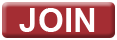SB184 (Castle Doctrine) - How They Voted

On May 28, 2008, the Ohio House of Representatives passed Amended SB184 (Castle Doctrine) with a strong bi-partisan majority. Here is how they voted.

Yeas - 73

Aslanides (R-94)
Bacon (R-21)
Batchelder (R-69)
Blessing (R-29)
Bolon (D-01)
Book (D-89)
Brinkman (R-34)
Carmichael (R-03)
Coley (R-55)
Collier (R-90)
Combs (R- 54)
Core (R-83)
Daniels (R-86)
DeBose (D-12)
DeWine (R-70)
Dodd (D-91)
Dolan (R-99)
Domenick (D-95)
Driehaus (D-31)
Dyer (D-43)
Evans (R-87)
Fende (D-62)
Fessler (R-79)
Flowers (R-19)
Gardner (R-06)
Garrison (D-93)
Gerberry (D-59)
Gibbs (R-97)
Goodwin (R-74)
Goyal (D-73)
Hagan J. (R-50)
Harwood (D-65)
Heydinger (D-58)
Hite (R-76)
Hottinger (R-71)
Huffman (R-04)
Hughes (R-22)
Husted (R-37)
Jones (R-67)
Lundy (D-57)
Mandel (R-17)
McGregor J. (R-20)
McGregor R. (R-72)
Mecklenborg (R-30)
Miller (D-10)
Newcomb (D-99)
Oelslager (R-51)
Okey (D-61)
Otterman J. (D-45)
Patton (R-18)
Peterson (R-2)
Raussen (R-28)
Redfern (D-80)
Reinhard (R-82)
Sayre (D-96)
Schindel (R-63)
Schlichter (R-85)
Schneider (R-35)
Sears (R-46)
Setzer (R-36)
Stewart J. (R-92)
Szollosi (D-49)
Uecker (R-66)
Wachtmann (R-75)
Wagner (R-81)
Webster (R-53)
White (R-38)
Widener (R-84)
Wolpert (R-23)
Yuko (D-7)
Zehringer (R-77)

Nays - 23

Beatty (D-27)
Boyd (D-9)
Brown (D-48)
Budish (D-8)
Celeste (D-24)
Chandler (D-68)
DeGeeter (D-15)
Foley (D-14)
Heard (D-26)
Koziura (D-56)
Letson (D-64)
Luckie (D-39)
Mallory (D-32)
Skindell (D-13)
Slesnick (D-52)
Stebelton (R-05)
Stewart D. (D-25)
Strahorn (D-40)
Sykes (D-44)
Ujvagi (D-47)
Williams B. (D-41)
Williams S. (D-11)
Yates (D-33)

On May 29, 2008, a strong bi-partisan majority in the Ohio Senate concurred with House amendments. Here is how they voted.

Yeas - 25
Amstutz (R-22)
Austria (R-10)
Boccieri (D-33)
Buehrer (R-01)
Cafaro (D-32)
Carey (R-17)
Cates (R-04)
Coughlin (R-27)
Faber (R-12)
Fedor (D-11)
Goodman (R-03)
Grendell (R-18)
Harris (R-19)
Jacobson (R-06)
Mumper (R-26)
Niehaus (R-14)
Roberts (D-05)
Schaffer (R-31)
Schuler (R-7)
Schuring (R-29)
Seitz (R-8)
Stivers (R-16)
Wagoner (R-2)
Wilson (D-30)

Nays - 7
Kearney (D-09)
Mason (D-25)
Miller D. (D-23)
Miller R. (D-15)
Morano (D-13)
Sawyer (D-28)
Smith (D-21)